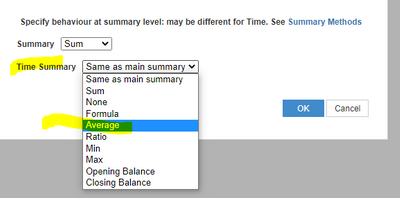# How to find monthly average of Daily Totals?I want to create the average of every month of a LI that displays Daily totals. One of the LI's will be my total account balances on a daily bases, and then I would want to create another LI that would show me the average of those daily total for each month. How would I go about this?

Thank you

Tagged:

•DAYS() function returns numbered value of days which you can use to calculate avarage

https://help.anaplan.com/fc064281-7c00-456f-821f-a94aebc35144-DAYS

Also check another formula DAYSINMONTH()

https://help.anaplan.com/bd6910ee-1a50-43e7-8bc1-c672899149df

•@neg177 It depends on how you want to calculate the Average:

You need to calculate the Average considering always the number of the days of the month, even an account has data only for some particular days.

Or... you need to calculate the Average considering only the nr. of the days where the amounts are not zero.

For considering ALL nr. of days of a month in the Average you can use the proposed @KirillKuznetsov  solution.. or if use the Time Anaplan native dimension, you can setup a Line-item with  Daily data and setup for Time list the Summary as "Average".If you need to consider only the days that are not zero.... you will need to create a daily line-item "Nr. Days Not Zero" where to put 1 when "Source Line-item" is not zero ( with a formula like "Source Line-Item" / "Source Line-Item" )

The AVG Line Item will have a formula like =  "Source Line-Item" / "Nr Days not Zero"

Hope it helps

Alex